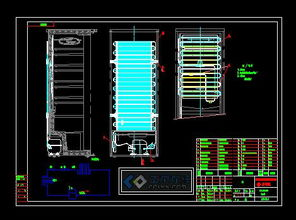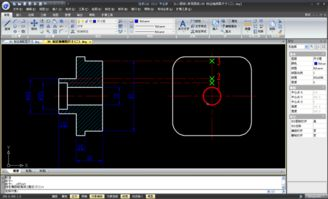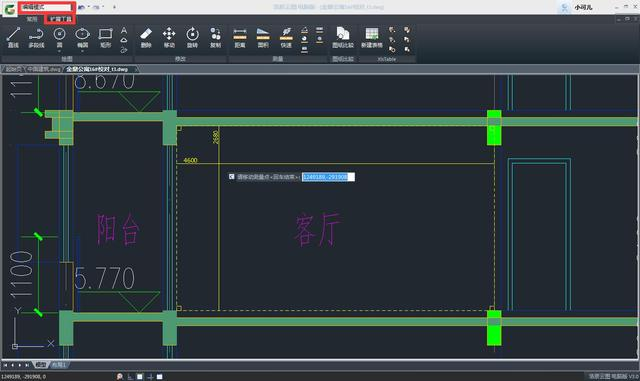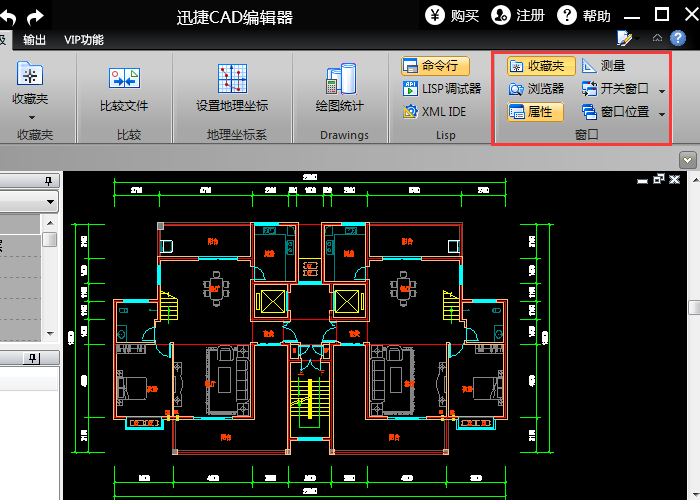33人浏览 / 0人评论L=直线；PL=多段线；U回车=Ctrl+z=后退； D=修改，调整； REC=矩形； C=圆； TR=修剪； O=偏移； XL=放射线； X=分解； CO=复制； M=移动； MI=镜像； EL=椭圆； BR=打断； POL=多边形； LEN=拉长； S=拉伸； ME=等分； E=删除； E回车ALL回车=全部删除； AR=阵列； RO=旋转； SC=比例缩放； END=端点； MID=中点； PER=垂足； INT=交足； CEN=圆心； QUA=象限点； TAN=切点； SPL=曲线； DIV=块等分； PE=编辑多边线； NOD=节点； F=圆角； CHA=倒角； ST=文字样式； DT=单行文字； T=多行文字； ED=编辑文字； A=弧； H =填充； HE=编辑填充； B=创建临时图块； W=创建永久图块； I=插入图块； LA=图层； MA=吸管加喷枪； （） PAR=平行线； FRO=正交

5、当我们在Word中点击取消这个图的组合的时候,会弹出一个对话框,询问你是否取消,这个时候就需要我们单击是,既可以成功的转换成WMF. 6、在做这些步骤的时候,建议可以多保存几张原图,以免在操作过程中不小心操作发生失误,这样我们就有可能要再次的制图,

------------------------------相关文章推荐 ------------------------------
[Image_Link]http://mie168.com/fileupload/08/pic/1275510897042187

[Image_Link]http://www.people.com.cn/mediafile/pic/20140922/83/36201476

小升初语文复习资料—词语

小升初语文复习资料—句子